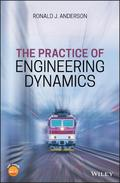John Wiley & Sons The Practice of Engineering DynamicsThe Practice of Engineering Dynamics is a textbook that takes a systematic approach to understanding.. Product #: 978-1-119-05370-5 Regular price: \$80.28 \$80.28 Auf Lager

# The Practice of Engineering Dynamics1. Auflage Juli 2020
240 Seiten, Hardcover
Wiley & Sons Ltd

ISBN: 978-1-119-05370-5
John Wiley & Sons

Jetzt kaufen

### Preis: 85,90 €

ca.-Preis

Preis inkl. MwSt, zzgl. Versand

The Practice of Engineering Dynamics is a textbook that takes a systematic approach to understanding dynamic analysis of mechanical systems. It comprehensively covers dynamic analysis of systems from equilibrium states to non-linear simulations and presents frequency analysis of experimental data. It divides the practice of engineering dynamics into three parts: Part 1 - Modelling: Deriving Equations of Motion; Part 2 - Simulation: Using the Equations of Motion; and Part 3- Experimental Frequency Domain Analysis. This approach fulfils the need to be able to derive the equations governing the motion of a system, to then use the equations to provide useful design information, and finally to be able to analyze experimental data measured on dynamic systems.

The Practice of Engineering Dynamics includes end of chapter exercises and is accompanied by a website hosting a solutions manual.

Preface xiii

1 Kinematics 3

1.1 Derivatives of Vectors 3

1.2 Performing Kinematic Analysis 8

1.3 2-D Motion with Constant Length 9

1.4 2-D Motion with Variable Length 14

1.5 Three Dimensional Kinematics 16

1.6 Absolute Angular Velocity and Acceleration 21

1.7 The General Acceleration Expression 23

Exercises 28

2 Newton's Equations of Motion

2.1 The Study of Motion 33

2.2 Newton's Laws 34

2.3 Newton's Second Law for a Particle 35

2.4 Deriving Equations of Motion for Particles 36

2.5 Working with Rigid Bodies 45

2.6 Using F = ma in the Rigid Body Force Balance 46

2.7 Using F = dG-- in the Rigid Body Force Balance 51

2.8 Moment Balance for a Rigid Body54

2.9 The Angular Momentum Vector - H O 59

2.10 A Physical Interpretation of Moments and Products of Inertia 65

2.11 Euler's Moment Equations 73

2.12 Throwing a Spiral76

2.13 A Two Body System77

2.14 Gyroscopic Motion 89

Exercises 97

3 Lagrange's Equations of Motion 101

3.1 An Example to Start 102

3.2 Lagrange's Equation for a Single Particle 106

3.3 Generalized Forces 114

3.4 Generalized Forces as Derivatives of Potential Energy 117

3.5 Dampers - Rayleigh's Dissipation Function 120

3.6 Kinetic Energy of a Free Rigid Body 123

3.7 A 2-D Example using Lagrange's equation 128

3.8 Standard Form of the Equations of Motion 133

Exercises 135

4 Equilibrium Solutions 139

4.1 The Simple Pendulum 139

4.2 Equilibrium with Two Degrees of Freedom 141

4.3 Equilibrium with Steady Motion 144

4.4 The General Equilibrium Solution 149

Exercises 150

5 Stability 153

5.1 Analytical Stability 154

5.2 Linearization of Functions 163

5.3 Example: A System with Two Degrees of Freedom 170

5.4 Routh Stability Criterion 177

5.5 Standard Procedure for Stability Analysis 183

Exercises 187

6 Mode Shapes 189

6.1 Eigenvectors 190

6.2 Comparing Translational and Rotational Degrees of Freedom 196

6.3 Nodal Points in Mode Shapes 207

6.4 Mode Shapes with Damping 208

6.5 Modal Damping 211

Exercises 218

7 Frequency Domain Analysis 223

7.1 Modeling Frequency Response 223

7.2 Seismic Disturbances 235

7.3 Power Spectral Density 238

7.3.1 Units of the PSD 248

7.3.2 Simulation using the PSD249

Exercises 256

8 Time Domain Solutions 259

8.0.1 Getting the Equations of Motion Ready for Time Domain Simulation 260

8.1 A time domain Example 262

8.2 Numerical Schemes for Solving the Equations of Motion 266

8.3 Euler Integration 267

8.4 An Example Using the Euler Integrator 272

8.5 The Central Difference Method - an O(h2) method 274

8.6 Variable Time-Step Methods 276

8.7 Methods with Higher Order Truncation Error 282

8.8 The Structure of a Simulation Program 285

Exercises 291

9 Experimental Data - Frequency Domain AnalysisF 295

9.1 Typical Test Data296

9.2 Transforming to the Frequency Domain - The CFT 298

9.3 Transforming to the Frequency Domain - The DFT 304

9.4 Transforming to the Frequency Domain - A Faster DFT 307

9.5 Transforming to the Frequency Domain - The FFT 309

9.6 Transforming to the Frequency Domain - An Example 310

9.7 Sampling and Aliasing 315

9.8 Leakage and Windowing 324

9.9 Decimating Data 328

9.10 Averaging DFTs 331

Exercises 332

A Representative Dynamic Systems 337

B Moments and Products of Inertia 361

B.1 Moments of Inertia 362

B.2 Parallel Axis Theorem for Moments of Inertia 364

B.3 Parallel Axis Theorem for Products of Inertia 367

B.4 Moments of Inertia for Commonly Encountered Bodies 368

C Dimensions and Units 369

D Least Squares Curve Fitting 373

Index
Ronald J. Anderson, Queen's University at Kingston, Canada
Dr. Anderson is a Professor in the Department of Mechanical and Materials Engineering at Queen's University at Kingston. He has been teaching courses and conducting research on the dynamics and vibrations of mechanical systems for over thirty years. In particular, his research has focused on ship motions, and road and rail vehicle dynamics.